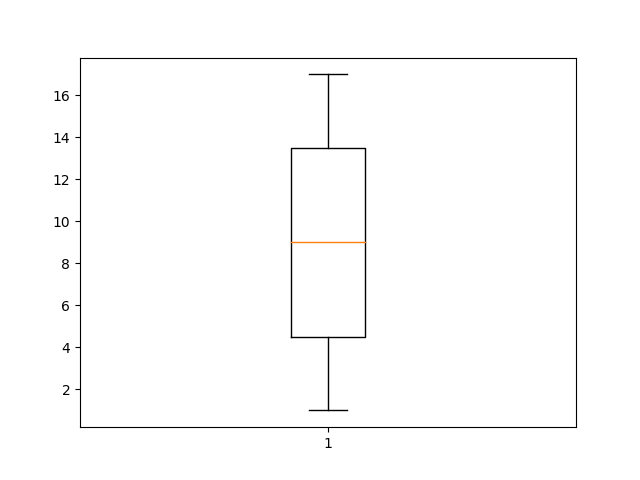Using the `numpy` library you can get various statistical values in Python. NumPy (Numerical Python) is a module consisting of multidimensional array objects and a collection of routines for processing those arrays.

On this page you learn how to apply statistical functions to a Python list. You don’t need advanced statistics knowledge to follow along.

Related Course:
Complete Python Programming Course & Exercises

## Five number summary

The five number summary is a set of functions in statistics that tell something about a data set. This includes the minimum, the maximum, the standard deviation, the mean and the median.

Import the `numpy` module.

You can optionally import the math module. Then create a list of numbers. In this example the list is defined manually, but of course you can load a list of numbers from excel, from a text file or another data source.

We can get the five number summary using the math library.

The five number summary contains: minimum, maximum, median, mean and the standard deviation.

All of these functions are implemented in the numpy module, you can either output them to the screen or store them in a variable.

The example program outputs the five number summary for the given list.

## Boxplot

In statistics, a box plot or boxplot is a method to graphically show groups of numerical data through their quartiles.Matplotlib has all kinds of plots. One of them being a function to create a boxplot. This code will create a boxplot: